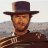# How do you create an indicator in ToS such that the BB is for the MACD?

T

#### tandeshao

##### New member
Hi I am using ToS and im trying to add Bollinger Bands to my MACD indicator. Does anyone knows how to do it?

Last edited:
T

#### tandeshao

##### New member
The calculation for the BB is taken from the MACD indicator instead of the stock's price

Last edited:#### BenTen

Staff
VIP
@tandeshao Try this code:

Rich (BB code):
``````declare lower;
#---------- MACD Inputs
input fastLength = 12;
input slowLength = 26;
input macdLength = 9;
input macdAverageType = AverageType.EXPONENTIAL;
#---------- BB Inputs
input displace = 0;
input length = 20;
input num_Dev_Dn = -2.0;
input num_Dev_up = 2.0;
input bbAverageType = AverageType.Simple;
#---------- MACD Section
def value = MovingAverage(macdAverageType, close, fastLength) - MovingAverage(macdAverageType, close, slowLength);
def avg = MovingAverage(macdAverageType, value, macdLength);
#---------- BB Section
def sDev = stdev(data = avg[-displace], length = length);
plot midLine = MovingAverage(bbAverageType, data = avg[-displace], length = length);
plot lowerBand = midLine + num_Dev_Dn * sDev;
plot upperBand = midLine + num_Dev_up * sDev;``````
Credit goes to Hahn.

Last edited:#### BenTenCreate a scanner for YungTrader's indicator? Questions 42